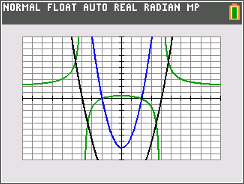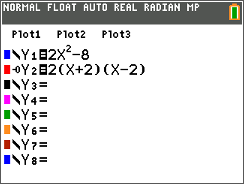•##### Download

•• ##### Device
• TI-84 Plus
• TI-84 Plus Silver Edition
•TI-84 Plus C Silver Edition
•TI-84 Plus CE
• ##### Report an Issue

Algebra II: Asymptotes and Zeros of Rational Functions
by Texas Instruments#### Overview

In this activity, students relate the graph of a rational function to the graphs of the polynomial functions of its numerator and denominator. Students graph these polynomials one at a time and identify their y-intercepts and zeros.

#### Key Steps

•In this activity, students relate the graph of a rational function to the graphs of the polynomial functions of its numerator and denominator. Students are first introduced to the definition of a rational function.

•Using the zeros found, students should write the factored form of y = 2x2 – 8 and enter it in Y2.

•If students graph the functions of the numerator, denominator, and rational function at the same time, they will see that the parabola of the numerator intersect at the rational function’s zeros and the parabola of the denominator intersects at the rational function’s vertical asymptotes.